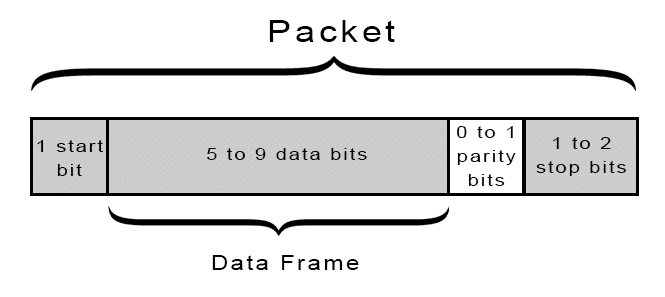# UART协议及其Verilog实现## 数据包的构成

Uart协议的传输数据被整合成数据包，每个数据包包含1位起始位，5-9位的数据位（具体决定于需求等因素），1位可选的奇偶校验位和1-2位的停止位。如下图所示：## 波特率

### 波特率和比特率

$I=S \cdot \log _{2} N$

$I=S \cdot \log _{2} N=9600 \cdot \log _{2} 2=9600 b i t / s$

### 常见波特率

9600、19200 、38400 、57600 、115200、230400、460800、921600

### 时钟与波特率的计算

FPGA 主频如果为50M，则时钟周期就是20ns。若数据发送速率为9600bps，则一位数据需要的时间为1000000000/9600=104167ns，则FPGA 传送一位需要翻转104167/20=5208个周期才可传送一位，所以程序中需计数5208才可满足9600bps。

## Verilog模块详解

### tx_clk_gen.v

timescale 1ns / 1psmodule tx_clk_gen#( parameterCLK_FREQUENCE= 50_000_000, //hz BAUD_RATE = 9600  //9600、19200 、38400 、57600 、115200、230400、460800、921600)( input clk,//system_clk input rst_n ,//system_reset input tx_done  ,//once_tx_done input tx_start ,//once_tx_start output  reg bps_clk//baud_rate_clk);localparam  BPS_CNT =CLK_FREQUENCE/BAUD_RATE-1,BPS_WD  =log2(BPS_CNT);reg [BPS_WD-1:0] count;reg c_state;reg n_state;//FSM-11'b0:IDLE1'b1:send_dataalways @(posedge clk or negedge rst_n) begin if (!rst_n)  c_state <= 1'b0; else  c_state <= n_state;end//FSM-2always @(*) begin case (c_state)  1'b0: n_state = tx_start ? 1'b1 : 1'b0;  1'b1: n_state = tx_done ? 1'b0 : 1'b1;  default: n_state = 1'b0; endcaseend//FSM-3 FSM's output(count_en) is equal to c_state//baud_rate_clk_counteralways @(posedge clk or negedge rst_n) begin if (!rst_n)  count <= {BPS_WD{1'b0}}; else if (!c_state)  count <= {BPS_WD{1'b0}}; else begin  if (count == BPS_CNT) count <= {BPS_WD{1'b0}};  elsecount <= count1'b1; endend//baud_rate_clk_outputalways @(posedge clk or negedge rst_n) begin if (!rst_n)  bps_clk <= 1'b0; else if (count == 'd1)  bps_clk <= 1'b1; else  bps_clk <= 1'b0;end//get_the_width_of_function integer log2(input integer v);  begin log2=0; while(v>>log2) log2=log2 1;  endendfunctionendmodule

### uart_frame_tx.v

timescale 1ns / 1psmodule uart_frame_tx#( parameterCLK_FREQUENCE= 50_000_000, //hz BAUD_RATE = 9600, //9600、19200 、38400 、57600 、115200、230400、460800、921600 PARITY = “NONE“ , //“NONE“,“EVEN“,“ODD“ FRAME_WD  = 8  //if PARITY=“NONE“,it can be 5~9;else 5~8)( input  clk,//system_clk input  rst_n ,//system_reset input  frame_en ,//once_tx_start input [FRAME_WD-1:0]  data_frame  ,//data_to_tx output  reg  tx_done  ,//once_tx_done output  reg  uart_tx//uart_tx_data);wire bps_clk;tx_clk_gen#( .CLK_FREQUENCE  (CLK_FREQUENCE),  //hz .BAUD_RATE(BAUD_RATE  )  //9600、19200 、38400 、57600 、115200、230400、460800、921600)tx_clk_gen_inst( .clk( clk  ),  //system_clk .rst_n ( rst_n),  //system_reset .tx_done  ( tx_done ),  //once_tx_done .tx_start ( frame_en),  //once_tx_start .bps_clk  ( bps_clk )//baud_rate_clk);localparam  IDLE  =6'b00_0000  ,READY =6'b00_0001  ,START_BIT=6'b00_0010  ,SHIFT_PRO=6'b00_0100  ,PARITY_BIT  =6'b00_1000  ,STOP_BIT =6'b01_0000  ,DONE  =6'b10_0000  ;wire [1:0]verify_mode;generate if (PARITY == “ODD“)  assign verify_mode = 2'b01; else if (PARITY == “EVEN“)  assign verify_mode = 2'b10; else  assign verify_mode = 2'b00;endgeneratereg  [FRAME_WD-1:0]  data_reg ;reg  [log2(FRAME_WD-1)-1:0] cnt  ;regparity_even ;reg  [5:0]  cstate;reg  [5:0]  nstate;always @(posedge clk or negedge rst_n) begin if (!rst_n)  cnt <= 'd0; else if (cstate == SHIFT_PRO & bps_clk == 1'b1)   if (cnt == FRAME_WD-1)cnt <= 'd0;  elsecnt <= cnt1'b1; else  cnt <= cnt;end//FSM-1always @(posedge clk or negedge rst_n) begin if (!rst_n)  cstate <= IDLE; else  cstate <= nstate;end//FSM-2always @(*) begin case (cstate)  IDLE  : nstate = frame_en ? READY : IDLE  ;  READY : nstate = (bps_clk == 1'b1) ? START_BIT : READY;  START_BIT: nstate = (bps_clk == 1'b1) ? SHIFT_PRO : START_BIT;  SHIFT_PRO: nstate = (cnt == FRAME_WD-1 & bps_clk == 1'b1) ? PARITY_BIT : SHIFT_PRO;  PARITY_BIT  : nstate = (bps_clk == 1'b1) ? STOP_BIT : PARITY_BIT;  STOP_BIT : nstate = (bps_clk == 1'b1) ? DONE : STOP_BIT;  DONE  : nstate = IDLE;  default  : nstate = IDLE; endcaseend//FSM-3always @(posedge clk or negedge rst_n) begin if (!rst_n) begin  data_reg <= 'd0;  uart_tx <= 1'b1;  tx_done <= 1'b0;  parity_even <= 1'b0; end else begin  case (nstate)IDLE  : begin
源文地址：https://www.guoxiongfei.cn/cntech/19303.html



 文章标签：UART协议及其Verilog实现 0
 猜你喜欢HTTP协议及其相关UART协议及其Verilog实现二进制协议 VS 文本协议数据库事务的概念及其实现原理维吉尼亚密码加解密原理及其实现【TCP协议】（1）---TCP协议详解WebSocket协议入门介绍RPC原理及其调用过程 ZAB协议简介 ZAB协议简介简单谈谈DNS协议RabbitMQ中RPC的实现及其通信机制WebSocket协议详解与c &amp;c#实现 python 迭代器协议和生成器 python 迭代器协议和生成器网络协议 19 - RPC协议综述：远在天边近在眼前Tomcat 对 HTTP 协议的实现（上）网络协议 12 - HTTP 协议：常用而不简单Tomcat 对 HTTP 协议的实现（下）网络协议 终章 - GTP 协议：复杂的移动网络 网络协议 20 - RPC 协议（上）- 基于XML的SOAP协议 网络协议 20 - RPC 协议（上）- 基于XML的SOAP协议【TCP协议】(3)---TCP粘包黏包网络协议 15 - P2P 协议：小种子大学问网络协议 22 - RPC 协议（下）- 二进制类 RPC 协议OPC协议解析-关于OPC协议的几个问题Java网络编程-HTTP协议 网络协议 21 - RPC 协议（中）- 基于 JSON 的 RESTful 接口协议 网络协议 21 - RPC 协议（中）- 基于 JSON 的 RESTful 接口协议网络协议 16 - DNS 协议：网络世界的地址簿使用Peach简化Socket网络通讯协议开发 Python：多态、协议和鸭子类型 Python：多态、协议和鸭子类型SMB协议原理抓包分析Swoole 多协议 多端口 的应用


 Twitter订阅我 声明：本站部分素材取自互联网，如有侵权，请发邮件到409840063@qq.com多谢！©2012-2019 郭雄飞 All Rights Reserved By www.guoxiongfei.cn var sl = 'https://www.cnblogs.com/halftop/p/verilog_UART.html'; document.getElementById("sourceUrl").innerHTML = sl; var _hmt = _hmt || []; (function () {var hm = document.createElement("script");hm.src = "https://hm.baidu.com/hm.js?fedf43e43a3df60f7196571653ecc070";var s = document.getElementsByTagName("script");s.parentNode.insertBefore(hm, s); })(); $(function () {$.each($("body a"), function (i, obj) { if ($(obj).html().indexOf("站长统计") > -1) { $(obj).html(""); }});$(".posttime a").attr("href", "#sourceUrl"); });